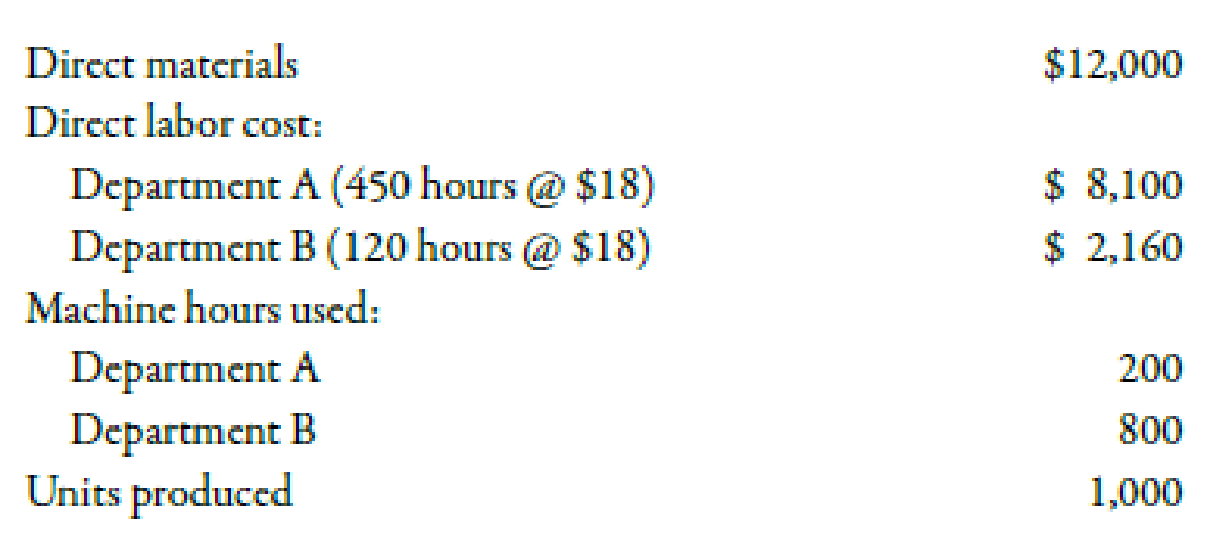Chapter 4, Problem 48E### Managerial Accounting: The Corners...

7th Edition
Maryanne M. Mowen + 2 others
ISBN: 9781337115773

#### Solutions

Chapter
Section### Managerial Accounting: The Corners...

7th Edition
Maryanne M. Mowen + 2 others
ISBN: 9781337115773
Textbook Problem
46 views

# Job Cost FlowsRoseler Company uses a normal job-order costing system. The company has two departments through which most jobs pass. Overhead is applied using a plantwide overhead rate of $10 per direct labor hour. During the year, several jobs were completed. Data pertaining to one such job, Job 9-601, follow:Required: 1. Compute the total cost of Job 9-601. 2. Compute the per-unit manufacturing cost for Job 9-601. For Requirements 3 and 4, assume that Roseler uses departmental overhead rates. In Department A, overhead is applied at the rate of$3 per direct labor hour. In Department B, overhead is applied at the rate of $7 per machine hour. 3. Compute the total cost of Job 9-601. 4. Compute the per-unit manufacturing cost for Job 9-601. 1. To determine Calculate the total cost of Job 9-601. Explanation Total Cost: Cost can be defined as the cash and cash equivalent which is incurred against the products or its related services which will benefit the organization in the future. There are two types of costs that are direct and indirect costs. The summation of all the cost is known as total cost. Compute the total cost of Job 9-601:  Amount($) Amount($) Direct materials 12,000 Direct labor: Department A 8,100 Department B 2,160 10,260 Overhead applied 5,700 Total cost 27,960 Table (1) Therefore, the total cost of Job 9-601 is$27,960...

2.

To determine

Calculate per unit cost for Job 9-601.

3.

To determine

Calculate the total cost of Job 9-601.

4.

To determine

Calculate per unit cost for Job 9-601.

### Still sussing out bartleby?

Check out a sample textbook solution.

See a sample solution

#### The Solution to Your Study Problems

Bartleby provides explanations to thousands of textbook problems written by our experts, many with advanced degrees!

Get Started